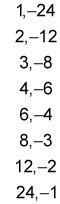##### GED Test: 1,001 Practice Questions For DummiesQuadratic equation problems on the GED Math test will generally require you to isolate the variable (usually x) and then solve for it. Some questions may just ask you to find the factored equation.

## Practice Questions

1. If x2 + 3x = 10, which of the following is a possible value of x?

A. –2 B. 5 C. 7 D. –5

2. Which of the following shows the correct factorization of the quadratic equation x2 – 2x – 24 = 0?

A. (x – 4)(x + 6) = 0 B. (x + 4)(x – 6) = 0 C. (x + 2)(x – 12) = 0 D. (x + 3)(x – 8) = 0

1. The correct answer is D.

You can solve the quadratic equation by factoring. Subtract 10 from both sides to set the equation equal to zero: x2 + 3x – 10 = 0

Factoring the equation gives you (x – 2)(x + 5) = 0. For the answer to be zero, either (x – 2) or (x + 5) has to equal zero (because any number times zero equals zero). Set each set of parentheses equal to zero and solve for x to find the solutions: (x – 2) = 0 gives you x = 2, and (x + 5) = 0 gives you x = –5. Hence, Choice (D) is correct.

2. The correct answer is B.

First, write the left-hand side of the equation as the product of two pairs of parentheses with x as the leading term:

(x)(x) = 0

Then list all the possible pairs of factors of –24:Now select the pair of factors that add up to the coefficient of the x-term in the equation, –2. You can save some time in this step if you apply some logic. The last term of the given equation is negative, so you know that one factor is positive and the other is negative. The factors need to have a difference of 2, so look at factors of 24 that are close together — 4 and 6 are only 2 units apart. Because the middle term is negative, the larger factor, 6, must be the negative one.

Substitute each value into the two pairs of parentheses:

(x + 4)(x – 6) = 0

An alternative method involves using FOIL (first terms, outer terms, inner terms, last terms) on each answer choice to see which one is equal to the original quadratic equation. Only Choice (B) gives you the original equation, so Choice (B) is the correct answer.